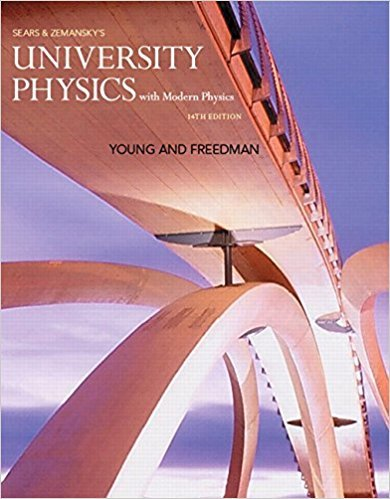×
Log in to StudySoup
Get Full Access to University Physics With Modern Physics (1) - 14 Edition - Chapter 34 - Problem Q34.2
Join StudySoup for FREE
Get Full Access to University Physics With Modern Physics (1) - 14 Edition - Chapter 34 - Problem Q34.2

Already have an account? Login here
×
Reset your password

# Answer: For the situation shown in Fig. 34.3, is the imageISBN: 9780321973610 228

## Solution for problem Q34.2 Chapter 34

University Physics with Modern Physics (1) | 14th Edition

• Textbook Solutions
• 2901 Step-by-step solutions solved by professors and subject experts
• Get 24/7 help from StudySoup virtual teaching assistantsUniversity Physics with Modern Physics (1) | 14th Edition

4 5 1 401 Reviews
22
0
Problem Q34.2

For the situation shown in Fig. 34.3, is the image distance s positive or negative? Is the image real or virtual? Explain your answers

Step-by-Step Solution:
Step 1 of 3

1 Friday, November 13, 15 Diffraction and interference 2 Constructive Interference (1) § The geometric optics of the previous chapter cannot be used to explain interference § To understand these interference + phenomena we must take into account the wave nature of light § Interference takes place when light waves of the same wavelength are = superimposed § If the light waves are in phase, they interfere constructively, as shown to the right 14 Constructive Interference (2) § The statement that the two waves interfere constructively is the same as saying that

Step 2 of 3

Step 3 of 3

##### ISBN: 9780321973610

The answer to “For the situation shown in Fig. 34.3, is the image distance s positive or negative? Is the image real or virtual? Explain your answers” is broken down into a number of easy to follow steps, and 24 words. The full step-by-step solution to problem: Q34.2 from chapter: 34 was answered by , our top Physics solution expert on 01/09/18, 07:46PM. Since the solution to Q34.2 from 34 chapter was answered, more than 246 students have viewed the full step-by-step answer. This full solution covers the following key subjects: . This expansive textbook survival guide covers 44 chapters, and 4574 solutions. This textbook survival guide was created for the textbook: University Physics with Modern Physics (1), edition: 14. University Physics with Modern Physics (1) was written by and is associated to the ISBN: 9780321973610.

Unlock Textbook Solution

Enter your email below to unlock your verified solution to:

Answer: For the situation shown in Fig. 34.3, is the image